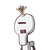# Rohan takes 6 days less than Rahul to finish a piece of work. If both Rohan and Rahul together can finish the work in 4 days, find

Rohan takes 6 days less than Rahul to finish a piece of work. If both Rohan and Rahul together can finish the work in 4 days, find the time taken by Rahul to finish the work

### 1 thought on “Rohan takes 6 days less than Rahul to finish a piece of work. If both Rohan and Rahul together can finish the work in 4 days, find”

1.x=12

Step-by-step explanation:

suppose B alone takes x days to finish the work .then A alone can finish it in (x-6)days

Now A’s one days wprks +Bs one days work

=1/x+1/x-6

and (A+B)one days work =1/4

x-6=1/4

x-6+x/x(x-6)=1/4=2x-6/x^2-6x=1/4

8x-24=x^2=6x

x^2-14x+24=0

x^2-122x+24=0

(x-12)(x-2)=0

x=12or x=2

but x cannot be less than 6 so x =12